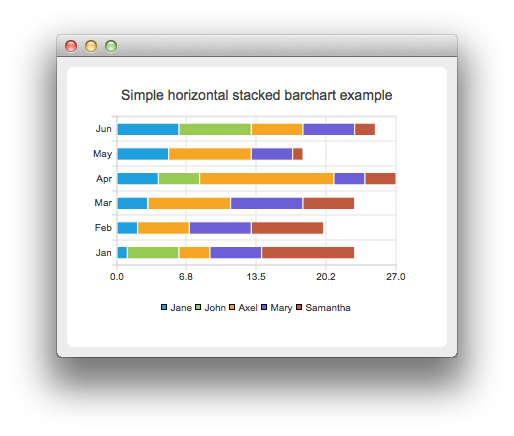### Find the Best Demos and Examples

Check out this quick tour to find the best demos and examples for you, and to see how the Felgo SDK can help you to develop your next app or game!

# HorizontalStackedBarChart Example

The example shows how to create a simple stacked horizontal bar chart.

The stacked bar chart shows the data in sets as bars that are stacked on top of each other. The stacking is done per category. Creating a stacked horizontal bar chart is just like creating a regular bar chart, except that for a stacked horizontal bar chart, we use the QHorizontalStackedBarSeries api instead of QBarSeries.## Running the Example

To run the example from Qt Creator, open the Welcome mode and select the example from Examples. For more information, visit Building and Running an Example.

## Creating Horizontal Stacked Bar Charts

The bar sets are used in same way in all bar charts. To illustrate the difference between various barcharts, we use the same data in all examples. The data that barchart visualizes, is defined by the QBarSet instances. Here we create the sets and append data to them. The data is appended here with the << operator. Alternatively, the append method could also be used.

```    QBarSet *set0 = new QBarSet("Jane");
QBarSet *set1 = new QBarSet("John");
QBarSet *set2 = new QBarSet("Axel");
QBarSet *set3 = new QBarSet("Mary");
QBarSet *set4 = new QBarSet("Samantha");

*set0 << 1 << 2 << 3 << 4 << 5 << 6;
*set1 << 5 << 0 << 0 << 4 << 0 << 7;
*set2 << 3 << 5 << 8 << 13 << 8 << 5;
*set3 << 5 << 6 << 7 << 3 << 4 << 5;
*set4 << 9 << 7 << 5 << 3 << 1 << 2;```

We create the series and append the bar sets to it. The series takes ownership of the bar sets. The series groups the data from sets to categories. The first values of each set are grouped together in the first category, the second values in the second category and so on.

```    QHorizontalStackedBarSeries *series = new QHorizontalStackedBarSeries();
series->append(set0);
series->append(set1);
series->append(set2);
series->append(set3);
series->append(set4);```

Here we create the chart object and add the series to it. We set the title for the chart with setTitle, and then turn on the animations of the series by calling setAnimationOptions(QChart::SeriesAnimations).

```    QChart *chart = new QChart();
chart->setTitle("Simple horizontal stacked barchart example");
chart->setAnimationOptions(QChart::SeriesAnimations);```

To have categories displayed on an axis, we need to create a QBarCategoryAxis. Here we create a category axis with a list of categories and set it to be the y-axis of the chart. The chart takes ownership of the axis. For the x-axis we use the default axis, which is created and scaled to series data by calling createDefaultAxes of the chart. Note that the call for createDefaultAxes must be before we set the category axis. Otherwise the default axis will override the category axis.

```    QStringList categories;
categories << "Jan" << "Feb" << "Mar" << "Apr" << "May" << "Jun";
QBarCategoryAxis *axis = new QBarCategoryAxis();
axis->append(categories);
chart->createDefaultAxes();
chart->setAxisY(axis, series);```

We also want to show the legend. To do that, we get the legend pointer from the chart and set it to visible. We also place the legend to the bottom of the chart by setting its alignment to Qt::AlignBottom.

```    chart->legend()->setVisible(true);
chart->legend()->setAlignment(Qt::AlignBottom);```

Finally we add the chart onto a view. We also turn on the antialiasing for the chartView.

```    QChartView *chartView = new QChartView(chart);
chartView->setRenderHint(QPainter::Antialiasing);```

The chart is ready to be shown. We set the chart to be the central widget of the window. We also set the size for the chart window and show it.

```    QMainWindow window;
window.setCentralWidget(chartView);
window.resize(420, 300);
window.show();```

Files: